# Variation of first derivative test for discontinuous function with one-sided limits

This article describes a test that can be used to determine whether a point in the domain of a function gives a point of local, endpoint, or absolute (global) maximum or minimum of the function, and/or to narrow down the possibilities for points where such maxima or minima occur.
View a complete list of such tests
This article describes a variation of first derivative test that is intended to remedy a specific defect, namely first derivative test fails for function that is discontinuous at the critical point.

## Statement

Suppose$f$ is a function and$c$ is a point in the domain of$f$. This statement is a variation of the first derivative test that helps deal with situations where a function has one-sided limits but is not necessarily continuous at the critical point of interest. It can be viewed as a remedy for the fact that the first derivative test fails for function that is discontinuous at the critical point.

### One-sided version

Limit existence assumption Comparison of one-sided limit and value Conclusion
Left hand limit of$f$ at$c$ exists Left hand limit is less than the value, i.e.,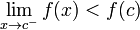$\lim_{x \to c^-} f(x) < f(c)$$f$ has a strict local maximum from the left at$c$, regardless of the way$f'$ behaves on the left of$c$
Left hand limit of$f$ at$c$ exists Left hand limit is greater than the value, i.e.,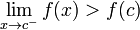$\lim_{x \to c^-} f(x) > f(c)$$f$ has a strict local minimum from the left at$c$, regardless of the way$f'$ behaves on the left of$c$
Left hand limit of$f$ at$c$ exists Left hand limit equals value, i.e.,$\lim_{x \to c^-} f(x) = f(c)$, so$f$ is left continuous We can try to use the one-sided version of the first derivative test:
If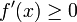$f'(x) \ge 0$ on the immediate left, then local maximum from the left
If$f'(x) \le 0$ on the immediate left, then local minimum from the left.
Right hand limit of$f$ at$c$ exists Right hand limit is less than the value, i.e.,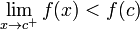$\lim_{x \to c^+} f(x) < f(c)$$f$ has a strict local maximum from the right at$c$, regardless of the way$f'$ behaves on the right of$c$
Right hand limit of$f$ at$c$ exists Right hand limit is greater than the value, i.e.,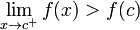$\lim_{x \to c^+} f(x) > f(c)$$f$ has a strict local minimum from the right at$c$, regardless of the way$f'$ behaves on the right of$c$
Right hand limit of$f$ at$c$ exists Right hand limit equals value, i.e.,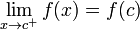$\lim_{x \to c^+} f(x) = f(c)$, so$f$ is right continuous We can try to use the one-sided version of the first derivative test:
If$f'(x) \ge 0$ on the immediate right, then local minimum from the right
If$f'(x) \le 0$ on the immediate right, then local maximum from the right.

### Two-sided version

We list the strict cases:

Case for left side behavior Case for right side behavior Conclusion for behavior of$f$ at$c$
Either$\lim_{x \to c^-} f(x) < f(c)$ or ($f$ is left continuous at$c$ and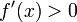$f'(x) > 0$ for$x$ on the immediate left of$c$) Either$\lim_{x \to c^+} f(x) < f(c)$ or ($f$ is right continuous at$c$ and$f'(x) < 0$ for$x$ on the immediate right of$c$) strict local maximum
Either$\lim_{x \to c^-} f(x) > f(c)$ or ($f$ is left continuous at$c$ and$f'(x) < 0$ for$x$ on the immediate left of$c$) Either$\lim_{x \to c^+} f(x) > f(c)$ or ($f$ is right continuous at$c$ and$f'(x) > 0$ for$x$ on the immediate right of$c$) strict local minimum
Either$\lim_{x \to c^-} f(x) < f(c)$ or ($f$ is left continuous at$c$ and$f'(x) > 0$ for$x$ on the immediate left of$c$) ither$\lim_{x \to c^+} f(x) > f(c)$ or ($f$ is right continuous at$c$ and$f'(x) > 0$ for$x$ on the immediate right of$c$) neither local maximum nor local minimum
Either$\lim_{x \to c^-} f(x) > f(c)$ or ($f$ is left continuous at$c$ and$f'(x) < 0$ for$x$ on the immediate left of$c$) Either$\lim_{x \to c^+} f(x) < f(c)$ or ($f$ is right continuous at$c$ and$f'(x) < 0$ for$x$ on the immediate right of$c$) neither local maximum nor local minimum